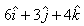NEET  >  Test: Gauss Law

# Test: Gauss Law

Test Description

## 10 Questions MCQ Test | Test: Gauss Law

Test: Gauss Law for NEET 2023 is part of NEET preparation. The Test: Gauss Law questions and answers have been prepared according to the NEET exam syllabus.The Test: Gauss Law MCQs are made for NEET 2023 Exam. Find important definitions, questions, notes, meanings, examples, exercises, MCQs and online tests for Test: Gauss Law below.
Solutions of Test: Gauss Law questions in English are available as part of our course for NEET & Test: Gauss Law solutions in Hindi for NEET course. Download more important topics, notes, lectures and mock test series for NEET Exam by signing up for free. Attempt Test: Gauss Law | 10 questions in 10 minutes | Mock test for NEET preparation | Free important questions MCQ to study for NEET Exam | Download free PDF with solutions
 1 Crore+ students have signed up on EduRev. Have you?
Test: Gauss Law - Question 1

### The Gaussian surface for a point charge will be

Detailed Solution for Test: Gauss Law - Question 1

A point charge is single dimensional. The three dimensional imaginary enclosed surface of a point charge will be sphere.

Test: Gauss Law - Question 2

### At what point is the electric field intensity due to a uniformly charged spherical shell is maximum?

Detailed Solution for Test: Gauss Law - Question 2

This is because when a charge is given to a hollow sphere that is a spherical shell then all charges will reside on its surface and as we know that electric field is directly proportional to given charge . so according to this concept of gauss’s law we can say about this point.

Test: Gauss Law - Question 3

### A charge q is placed at the centre of the open end of cylindrical vessel whose height is equal to its radius. The electric flux of electric field of charge q through the surface of the vessel is

Detailed Solution for Test: Gauss Law - Question 3

Given that, A charge q is placed at the centre of open end Q a cylindrical vessel,we have to find the flux through the surface of the vessel.
so, when charge Q is placed at the centre of open end of a cylindrical vessel then only half of the charge will contribute to the flux, because half will lie inside the surface and half will lie outside the surface.
so, flux through the surface of vessel is q/2ε0

Test: Gauss Law - Question 4

Which of the following quantity is a scalar?

Detailed Solution for Test: Gauss Law - Question 4

Electric flux is a scalar quantity. It is a scalar because it is the dot product of two vector quantities, electric field and the perpendicular differential area.

Test: Gauss Law - Question 5

If an excess charge is placed on an isolated conductor, then, that amount of charge

Detailed Solution for Test: Gauss Law - Question 5

An isolated conductor is any metal object not connected to or not in contact with any other conductor . one of its common properties is that there is no charge of any nature within the surface of the conductor. All charge, if any, always resides on the outer surface only.thus , if we place any amount of charge, it would tend to reside on its surface.

Test: Gauss Law - Question 6

The charge enclosed by a spherical Gaussian surface is 8.85 X 10-8 C.What will be the electric flux through the Surface?

Detailed Solution for Test: Gauss Law - Question 6

we know that the flux linked with a Gaussian surface is given as
Φ = E.ds = q/ε0
here,
q = 8.85x10-8 C
ε0 = 8.85x10-12 F/m
so,
Φ = 8.85x10-8 / 8.85x10-12
thus, flux will be
Φ = 104 Nm2/C-1

Test: Gauss Law - Question 7

If the electric field is given by; calculate the electric flux through as surface of area 10 units lying in y-z plane.​

Detailed Solution for Test: Gauss Law - Question 7

As surface of area lies in the Y-Z plane, thus its area vector points in X direction i.e.   A=10i
Electric flux    ϕ=E.A
∴   ϕ=(6i+3j​+4k).(10i)
⟹   ϕ=6×10=60 unit

Test: Gauss Law - Question 8

The flux associated with a spherical surface is 104 N m2C-1.  What will be the flux, if the radius of the spherical shell is doubled?​

Detailed Solution for Test: Gauss Law - Question 8

If the radius of the spherical shell is double then also flux associated with spherical surface remain unchanged because the charge enclosed in system always remains unchanged.

Test: Gauss Law - Question 9

The Gaussian surface for a line charge will be

Detailed Solution for Test: Gauss Law - Question 9

A line charge can be visualized as a rod of electric charges. The three dimensional imaginary enclosed surface of a rod can be a cylinder.

Test: Gauss Law - Question 10

The electric field intensity due to a sphere (solid or hollow) at an external point varies as-​

Detailed Solution for Test: Gauss Law - Question 10

In case of a sphere (solid/hollow), electric intensity (E) at an external point varies as 1/r2, where r is the distance of the point from the centre of the sphere. In case of a line charge, E o 1/r and in case of an infinite plane sheet of charge, E does not depend upon r.

 Use Code STAYHOME200 and get INR 200 additional OFF Use Coupon Code
Information about Test: Gauss Law Page
In this test you can find the Exam questions for Test: Gauss Law solved & explained in the simplest way possible. Besides giving Questions and answers for Test: Gauss Law, EduRev gives you an ample number of Online tests for practice

### How to Prepare for NEET

Read our guide to prepare for NEET which is created by Toppers & the best Teachers